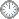# 顶级作品才是最好的老师~

This is an in-mail from TYUST.
新入の者--> What is going on ? (redirected)new

(接前：21 18 17) 温习: 命题5.7的证明.

Γv = (1 + t)B~ + (1 - eps/4)ΣEi + (1 - a)T.
---- 此表达式结构复杂，不会凭空而来.
---- 上回探明，从ψ*出发可得到Γv的“毛坯”:
(1 + t)B~ +  ΣEi + (1 - a)T.
---- 此式含有Γv的各项“元件”，只一处系数不同.
---- 系数 (1 - eps/4) 的起因值得探求(?).
.

1. 公式： ψ*(Kx + B) = Kv + B~ + s·Eψ.
---- s·Eψ 是所有配系数超常除子之和.
---- = (...,1-ai,...); Eψ = (...,Ei,...).

= (...,1-ai,...,1-a); Eψ = (...,Ei,...,T).
2. 两个“边界”(或“相”): B 及 B + tB (即 (1+t)B ).
---- B 是原有的; B + tB 是导出的(参后文).
3. 两个配对: (X, B) 以及 (X, B + tB).
---- 前者是 eps-lc, 后者是 eps/2-lc.
4. 计算：ψ*(Kx + B) 以及 ψ*(Kx +(1 + t)B).
(在计算中，配对表达为“运算形式”).
ψ*(Kx + B) = B~ + ΣEi + (1 - a)T - ΣaiEi.
ψ*(Kx +(1 + t)B) = (1 + t)B~ + ΣEi - Σa'iEi + (1 - a')T.
(集项的原则:剔除 ai,a'i, a'; 1-a' 看作整体).
5. 构造: 两式的粉色项取并集; 各项做和:
(1 + t)B~ +  ΣEi + (1 - a)T.
---- 此即为 Γv 的“毛坯”. 暂记 Uv.
6. 图解:
ψ*   (Th1.6)
.
X     B|B+tB

.

---- 粗略的判断，该是与 (V, Γv) klt 有关.
---- Step5 “By construction, (V, Γv) is klt”.
---- 但不是为了得到任意 klt 型的配对.
---- 特定地，原作要让 Γv 关联 B 和 B + tB.
---- 另外，Kv + Γv 有两种余项 tB~+F 及 G.
---- G 存活到Step5, 而 F 存活到Step6末.
---- 凑巧的是 Kv + Γv  G/X 有某种“整性”.
---- 这是让 Γv 关联 B + tB 的原因吗 ?
---- Step4第一段落点是(X, B + tB) is eps/2-lc.
---- 换句话说，原作特意准备了此配对.
---- 原作如何知道该做这个准备呢 ?
---- B + tB 与 B + 2tB 有关，后者源于Th1.6.
---- Th1.6 具体起到什么作用 ?

.

符号大全上下标.|| 常用：↑↓ π ΓΔΛΘΩμφΣ∈  ∪ ∩ ⊆ ⊇ ⊂ ⊃ ≤ ≥ ⌊ ⌋ ⌈ ⌉ ≠ ≡ ⁻⁰ ¹ ² ³ ᵈ ₀ ₁ ₂ ₃ ᵢ .

#### Glossary(AG)

*

Introduction
....
....
...

http://blog.sciencenet.cn/blog-315774-1175067.html

## 全部精选博文导读

GMT+8, 2019-7-16 22:31